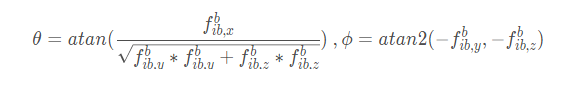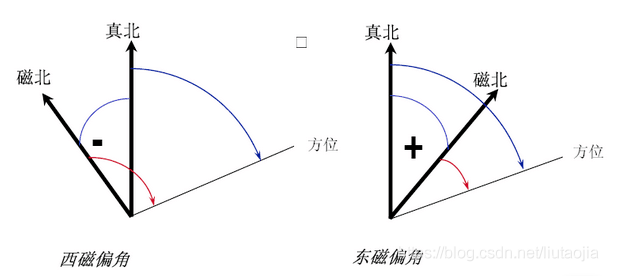Application

# Determination of Initial Attitude Angle of MEMS Inertial Sensor

With the continuous development of Micro Electromechanical System (MEMS) technology, MEMS-based inertial sensors have been widely used, such as robot tracking and navigation, and pedestrian positioning. The inertial navigation system uses the initial position, velocity and attitude information to obtain the position, velocity and attitude information of the target by quadratic integration of the angular rate and specific force measured by the gyroscope and acceleration. Therefore, the quality of the initial alignment will determine the accuracy and stability of the inertial navigation system.

The accuracy of the accelerometer in the MEMS inertial sensor is usually better than 1mg, and the gyroscope cannot be sensitive to the rotation of the earth, so the accelerometer can be used to determine the roll angle and pitch angle of the inertial navigation system, but the heading angle information cannot be determined by the gyroscope. The initial heading angle of the inertial navigation system can be determined using the magnetometer

The relationship between the navigation coordinate system and the carrier coordinate system

The navigation coordinate system here adopts the North-East-Earth (NED) coordinate system, denoted as the n system; the carrier coordinate system adopts the forward-right-down coordinate system, denoted as the b system. Rotation around the carrier’s x-axis is the roll angle, rotation around the carrier’s y-axis is the pitch angle, and rotation around the carrier’s z-axis is the heading angle. The navigation coordinate system can be transformed into the carrier coordinate system by rotating along the x-axis, y-axis and z-axis in turn, then the attitude rotation matrix DCM of the carrier coordinate system and the navigation coordinate system can be expressed as: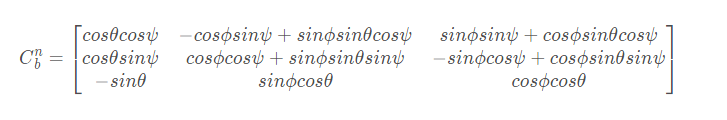Among them, θ is the pitch angle, ϕ is the roll angle, and ψ is the heading angle.

Note: The selected navigation coordinate system and carrier coordinate system are inconsistent with those here, and the rotation order is inconsistent with that here, resulting in different rotation matrices.

Note: The coordinate system and attitude rotation matrix are the most basic and core parts of the inertial navigation system.

Calculation of initial roll and pitch angles

When the sensor is stationary, the accelerometer measures the projection of gravity on the carrier coordinate system. Due to the large error of the MEMS sensor device, the projection of gravity in the north and east directions can be ignored, that is, the vertical deviation of gravity is not considered, then the relationship between gravity and the specific force (acceleration) of the accelerometer is: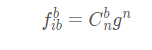Among them,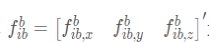is the specific force (or acceleration) information of the x, y and z axes output by the accelerometer, and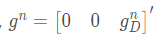is the projection of gravity in the north (North), east (East) and ground (Down) directions in the navigation coordinate system. Through the above DCM matrix, it can be expanded as: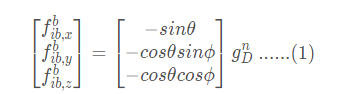The initial roll angle and pitch angle of the inertial sensor during stationary period can be calculated by formula (1). The calculation formula is: